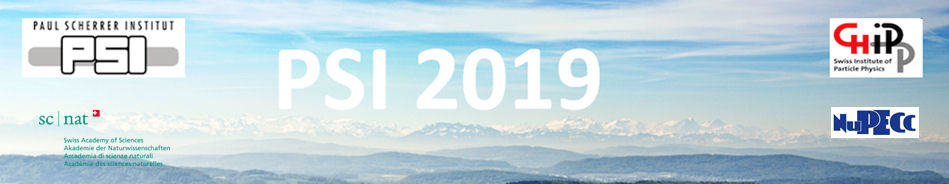#Physics of fundamental Symmetries and Interactions - PSI2019

Oct 20 – 25, 2019
PSI
Europe/Zurich timezone

## Test of the crystal-diffraction ultra-precise neutron spectrometry.

Oct 24, 2019, 12:00 PM
20m
WHGA/001 - Auditorium (PSI)

Oral

### Speaker

Prof. Vladimir Voronin (NRC "Kurchatov institute"-PNPI)

### Description

Recently proposed ultra-precise neutron spectrometry technique based on the effects of dynamic neutron Laue-diffraction in perfect crystals was experimentally tested.
This technique is based on the effect of enhancement of angular deviation of neutron trajectory inside the crystal when the angle of neutron incidence on the crystal is slightly varied. Moreover, there is an additional gain factor tan^2⁡(θ_B) that becomes significant in the case of large diffraction angles. This factor is due to the fact that the neutron propagation time inside the crystal is increased with increasing the diffraction angle. We propose to use these two effects of the angular amplification for studies of the impact of external forces that might influence diffracting neutrons.
Such a technique could be used for a broad range of experiments:
The accuracy of the neutron electric charge experiment can be improved by one order of magnitude compared with the current experimental limit;
A test of the equivalence of inertial and gravitational neutron masses can be done with an accuracy of 10^{-5};
Neutron scattering amplitudes can be measured with high accuracy both for solid materials and gases;
Neutron diffraction in perfect crystals and crystal properties on the inter-planar distance homogeneity about Δd/d ~(10^{-6} -10^{-8}) can be studied.
A double-crystal setup made of highly-perfect silicon crystals to test this technique was constructed and mounted on the PF1b beam at ILL reactor.
The factor of diffraction amplification of the beam splitting in a magnetic field gradient (analogy of Stern-Gerlach effect) was measured. This factor reaches 6 *10^{6} for the (220) silicon plane at Bragg angle equal 82^{0} in comparison with a free neutron.

### Primary author

Prof. Vladimir Voronin (NRC "Kurchatov institute"-PNPI)

### Presentation materials

 Voronin_Difr_PSI-2019_for pdf.pdf Voronin_Difr_PSI-2019_for pdf.ppt